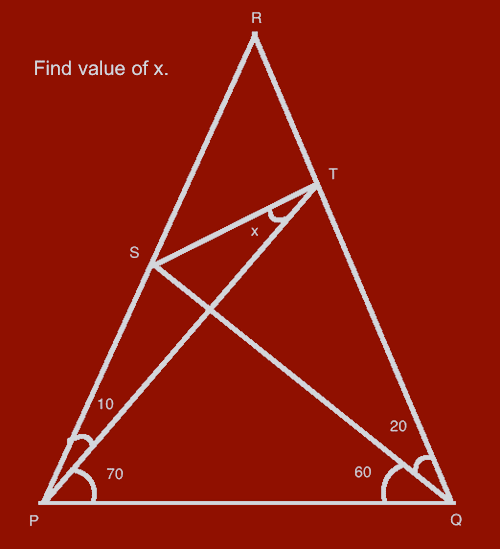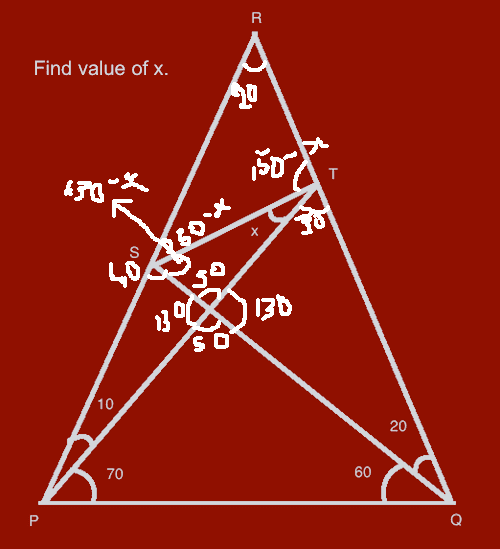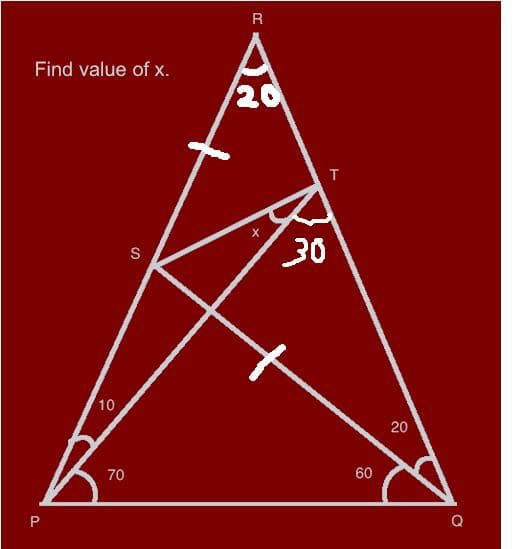# Grand Quiz Question #2

The 2nd Question of the #-Link-Snipped-# is as follows -

Q. Using basic geometry rules, find the value of 'x' in the following figure.
[Question is worth 15 CE-Coins]

You shall not use trigonometry rules such as sines and cosines.HINT: The image has not been drawn to proportion. It is advised that you draw the diagram yourself before solving.

A few instructions -

value of x along with the approach you used to solve this problem.
We expect you to give a logical explanation of how you reached to the solution.

2. Answers with no explanation at all will be disqualified.

3. Just as we said before, the fastest to answer correctly wins!

## Replies

•Anoop Mathew
x is 20 degrees. Exterior Angle is equal to interior opposite angle. angle ART is 20, so x also becomes 20
•Anoop Mathew
I meant angle SRT is 20, not ART...Typing mistake.
•PraveenKumar Purushothaman
The answer is 20 degrees. I drew it using hand and measured it. I can also be done as this way.
R = 100 = 80 + 80 + R.
P = 10.
Mid Pt = 180 - 60 - 70 = 130.
So, 150 - 130 = 20!
•Kaustubh Katdare
Do NOT make assumptions, folks 😀
•Sagar07
In triangle PQR, angle R=180-angle P- angle Q= 180- 80-80= 20....
angle RTS is 90..
hence in triangle RTS, angle RST=180- angle R- angle RTS=189-20-90=70
angle PSQ=180-10-90=80

hence angle TSQ=180- angle PSQ- angle RST=180-70-80=30

in triangle TSO, x= 180-90-angle TSQ= 180-90-30=60

assuming intersection point is o......
•Sahithi Pallavi
i can give you the explanation in this figure,
so by considering the middle triangle,
20+ (50-x)+ (150-x) = 180.
220-2x = 180.
40 =2x.
x=20!
•Sahithi Pallavi
sorry, forgot to add this image•bhavna gupta
Let the point inside the triangle be taken as A
In triangle PQR
According to the property 1:all the sum of all the angles of a triangle is 180 or 360
therefore, P+Q+R=180
80+80+R=180
R=20

In triangle RSQ
as above property.......R+Q+S=180
20+20+S=180
S=140

Now,acc to the property 2:sum of all the angles made across the line is 180
therefore,In triangle POS...
S=180-140
S=40

Again In triangle POS..
P+S+O=180
10+40+O=180
O=130
now In triangle POQ
P+Q+O=180
70+60+O=180
O=50

In triangle RST
R+S+T=180
20+140+T=180
T=20

now acc to property 2:
O in triangle SOT
130+O=180
O=50

acc to property 2:
O in triangle QOT
O=130

In triangle QOT
t=30
now acc to property 2: At T
T of triangle RST+T of triangle SOT+T of triangle QOT=180
20+T+30=180
•By Z property angle T=70, Given PTQ angle =90, and RTQ=180 so x=180-70-90=20.......
thats all.........
•Kaustubh Katdare
Repeat: DO NOT make assumptions, please! :😀
•CIVILPRINCESS
X is 60

the triangles RST AND QST are similar... since QS=SR (OPPOSITE ANGLES ARE 20) and they have a common side ST. (SSA congruency)

i.e. RTS = STQ. => 90

X+30 = 90. => X=60.
•CIVILPRINCESSTHE SIDEs I'VE marked (SQ and SR) are equal and one common side ST and one equal angle 20. so SAS congruency...
•ultimate_k
x is equal to 50 degree (trial and error method).
•Guneet Khanuja
x= 70 degree.
method: let angle TSQ= y, and angle RST= z.
therefore,
y+z=140
x+y=130
z-x=10.
and SR= SQ
•eternalthinker
Can't find a logical way to x!

Appreciate the question anyways 😀
•eternalthinker
The thumbnail was from previous attempts to answer. Auto-saved!
•lovejeet
x=30...
psq=180-spq-sqp=40------------A
angle sot(o is the intersection of sq and tp)=50---------------B
prq=20-----------------------C
in triangle pts, pst=140-------------D
THerefore, tsq=100...
Therefore, x=180-100-50=30
HENCE, X=30.
•abhinaykumar
by simple triangle method all angles in triangle= 180 using this equation we can solve the problem
as we all can see there are two rightangled triangles
using those we can say the angle of x is 20
•sunil pal
triangle STR&STQ
ST=ST
angle SQT=angle SRT
in triangle SQR SR=SQ
so angle RST=angle TSQ
angle RSQ=140=angle RST+angle TSQ
so angle RST = angle TSQ=70
in triangle SPQ
angle PSQ=40
and angle PTQ=30
and
X+angle PTQ=90
so
X=60 ANS
X=
•Ankita Katdare
Entries for this question are now closed.

Check out today's question between 6pm to 9pm IST.

You are reading an archived discussion.

## Hi All I am new to CE

hi This is Binod from India. I am so glad to find this and I like to make friends to share my thoughts. I am currently working in an IT...

## Samsung Galaxy Note Launched In India!

Samsung Galaxy Note has been launched in India for price Rs. 35000: Samsung Galaxy Note Price In India Is Rs. 34990 | Samsung Galaxy Note Talk

## help for project

please help me select the final year project on electronics and communication involving recent technology..preferably on computer networking😀

## How does the computer manage overflow? Explain with an example.

How does the computer manage overflow? Explain with an example. can anybody please explain me the concept in brief in simple way ?😔

## Explain about IEEE standard representation of floating point numbers

Explain about IEEE standard representation of floating point numbers😕